# Riemann prose, part 7

“i” represents a phase change. You’re rotating a point in the complex plane counterclockwise around the origin.

1
1 * i = 1i
1i * i = -1
-1 * i = -1i
-1i * i = 1

So, how does a phase change translate to being used as an exponent? That is, are we multiplying a number by itself “theta” degrees times?

x^bi = cos(ln(x^b)) + i*sin(ln(x^b))

Basically, we’re rotating the point, maybe?
What is “ln()”?

A logarithm is a conversion formula where

if y = b^x, then x = logb(y)

As an example,
y = 10^2 = 100
x = log10(100) = 2

Or, the log of a number using a particular base will give you the power using that base that gives you that number.

10 squared is 10*10 = 100. The log of 100, using base 10, is 2.
10 cubed is 10*10*10 = 1000. The log of 1000, using base 10, is 3.

Logarithms are useful because they let you draw huge exponential curves as straight lines on a smaller sheet of paper.
Note that as x goes from 1 to 100, log10(x) goes from 0 to 2.

Because our modern counting system is decimal-based, it’s natural to use log10() in many cases. However, we want ln(), which is called the natural logarithm.

There is a special number, e, that is defined as the sum of 1 over n factorial (the product of multiplying the integers between 1 and n). It was first defined as a constant by Jacob Bernoulli in the late 1600’s when he was studying compound interest.

The natural logarithm is “loge()”, or, “log base e”, written as “ln()”.

e has some special properties of its own, including Euler’s Identity: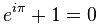Kinda cool, eh? It also means that e^i*pi = -1. Rotation, baby!

Going back to imaginary exponents:

x^bi = cos(ln(x^b)) + i*sin(ln(x^b))

This just means that instead of trying to calculate “x^bi”, we can convert it by taking the natural logarithm of x^b, and then plugging that into the sine and cosine wave formulas.

Oh, wait, I haven’t talked about those yet?(How triangles relate to circles.)

I mentioned earlier that a number in the complex plane can either be represented as the two legs of a right triangle, or as a vector in the form of the magnitude (hypotenuse of the triangle) and the angle theta. The cosine() of an angle extracts the real part of the magnitude of the vector (“a”), and the sine() of the angle extracts the imaginary part (“b”). That is, these are conversion factors to switch between rectangular and polar (circular) graphs.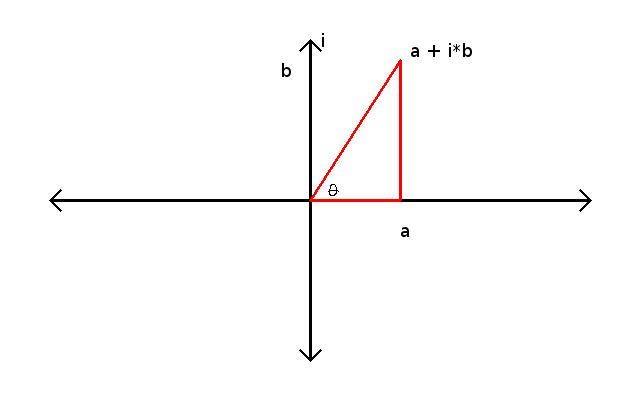(Note: Images takes from wikipedia.)

# Gakken Updates

With the year pretty much wrapping up, things are pretty quiet with Gakken. There were two posts on the Facebook page, one announcing the release of the Rainbow Loom for Adults kit, and the other talking about about a theremin demo at Tokyo Culture Culture in Shinjuku on Dec. 21st.

Other than that, there’s nothing to report on. Literally nothing, in that the Otona no Kagaku page hasn’t been updated to show the Next Up page, and Amazon doesn’t have a placeholder page for the next kit. The last mook advertised a “hurricane-style” humidifier, with no tentative release date. Since the Steel Drum kit came out at the end of September, with a big gap between it and the previous kit, I was hoping for a December-January date on the humidifier. That’s not likely right now, and I’m not expecting to see anything new from Gakken until maybe March, at this point.

# Riemann prose, part 6

Instead of treating the x-y graph as being a simple combination of a single-dimensional variable plus the result of the equation, what if we make x into two dimensions, and call the output of the equation “z”?

z = 2x + 3y

We’re no longer drawing a line; now we’re making a sheet, or plane. We want “x” and “y” to be independent of each other, and to have certain properties. We want to keep the scaling factor, so that “x” times x moves us left and right on the horizontal axis, and “x” times y moves us up and down on the vertical axis.

For “y”, we want something connected to that “-1” mentioned in the last blog entry. We want “y” times x to be xy, but “y” times y to be -1.

You probably already know where I’m going with this.

If we calculate the areas of each of the squares in the four quadrants:

50 * 50 = 2,500
50 * (-50) = -2,500
(-50) * 50 = -2,500
(-50) * (-50) = 2,500

We can get the areas in each case, but how do we work backwards from the area to get the length of the side for that square?

y = squareroot(2500) = +50, or -50
y = sqrt(2500) = +/-50

Ok, fine, this works. But,

y = sqrt(-2500)?

Fail. We don’t have a number that, times itself, equals -1.

Yet. But, this is exactly the connection to -1 that we’re looking for in our speculated vertical axis element, “y”. “y” * “y” = -1.

I’ll quit the drama, and say that we’re reached “i”. The purpose of “i” is to rotate a point counterclockwise around the origin (0,0).Because “i” doesn’t physically exist, it’s a bookkeeping placemarker, numbers that include “i”, in the form of “b*i”, were called “imaginary numbers”, while our existing numbers (“a”) were identified as “real numbers”. Combining them in the form of “a + i*b” creates complex numbers.

“a” and “i*b” are related by being the two sides of a right-angle triangle. We can either write a complex number as (1.3 + 2.7i), or as a vector – magnitude (mag) and angle. Where mag is sqrt(a^2 + b^2), and the angle (theta) is arctan(b/a).

2 * (a + ib) = 2a + 2ib (scaling)
i * (a + ib) = ia – b (rotation)
(a + ib)^2 = a^2 + 2iab – b^2 (both)

Complex numbers are symbolized as “s”. Now, where have we seen “s” before?Hmm. That looks like the Riemann Zeta function again. 1/n^2 could be rewritten as

1/n^(a+ib), where a = 2 and b = 0

How would that work?

Let’s backtrack and just use real numbers.

n^a = n * n * n *… “a” times
n^2 = n * n
n^3 = n * n * n
n^4 = n * n * n * n

Here’s an interesting thing: n*n*n*n*n = (n*n)*(n*n*n) = (n^2) * (n^3) = n^5

Exponents (the powers following the “^” symbol) of a specific value “n” are additive. So,

n^(a+ib) = (n^a) * (n^ib)

We already have an idea of what “n^a” does, as long as “a” is a positive integer. What if it’s NOT a positive integer?

1/n = n^(-1)

Having a negative real number in the exponent is just the same as taking the reciprocal of “n”.

n^(1/2) = sqrt(n)

If n squared (n^2 = n * n) = 2,500, then the value of n, which squared gives us 2,500, must be + or – 50. That is, n = 2500^(1/2) = +/-50.

A cube is defined as a*a*a = a^3,
so the cube root of a number will be the length of one side of that cube, “a”.

8^(1/3) = 2

We could also write n^1/2 as n^0.5

So, fractional values in the real portion of the exponent just refer to root operations (square root, cube root, fractional roots, etc). That is, for “n^2.5”, n is equal to:

n*n * extra

Where “extra” is whatever number gives you “n” when multiplied by itself 1/0.5 (2) times.

9^2.5 = 9^2 * 9^0.5 = 9*9 * sqrt(9) = 81 * 3 = 271

So, what do imaginary exponents do?

# Bokaro P ni Naritai, vol. 7(Images used for review purposes only.)

I want to be a Vocaloid Producer, vol. 7, 1,500 yen, plus tax.
The main premise of the Vocaloid P magazines is that Rana is attending Crea, a school for new Vocaloids (humanoid machines designed as music entertainment systems). In volume 1, Crea was presented as Rana’s new home, and a certain amount of page-space was dedicated to showing the design and floor plan. Since then, though, Crea only appeared in the 4-panel comics as the background setting for the jokes. Volume 7 spends a couple pages this time talking about the Warp Room, an area just off the main front lobby that students use to teleport to the different classroom wings.

As far as MikuMiku Dance is concerned, the Warp Room is another dance stage for the music videos. The DVD-ROM includes two new MMD accessory model files – the Warp Room, and a matching set of jewelry for Rana (red wavy star armlet, earrings and finger ring).

New magazine features:
The 4-panel comic showing Rana discovering the Warp Rooms for the first time. Robo-Panda teaching Rana about the different parts of a drum kit based on the sounds they make, and connecting that to the SSW MIDI drum kit voices. Interview with guitarist Jimmy Thumb P. Overview of the Galaco Vocaloid voice add-on. Pick-up artist: An.

New DVD Features:
Song Title: Romantic Distance (?), Artist: An
This time, we just get the song without an accompanying video, and since the name isn’t specified in the file, I’m guessing that it’s called “Romantic Distance” based on a single clue from the magazine. It’s a bouncy J-pop piece without anything really outstanding about it. Rana’s voice is kind of mechanical-sounding, like it wasn’t fully tweaked. It also suffers from a problem that many Japanese songs have – it doesn’t scan. Because the Japanese sentence structure doesn’t flow in the same way English does, there’s a tendency to cram too many syllables into one line to make a complete sentence.The Crea Warp Room stage, and Rana’s jewelry accessories.)

Tutorials:
Vocaloid:
The focus this time is on editing per-note properties. The last 2-3 lessons talked about parameters that could be applied throughout the song, including Velocity and Dynamics. Here, clicking on an individual Vocaloid phoneme brings up the Note Properties window, where you can choose between accent and vibrato. Accent can soften or emphasize the enunciation of the phoneme, which equates to syllable stress within a word in English. Accent also allows for Portamento for rising or falling parts of the notes. In this case, rather than Vocaloid jumping in pitch from one phoneme to the next, you can have the voice sweep smoothly between the notes, which is similar to what happens with Portamento on a synthesizer keyboard. Finally, we get Vibrato, the warbling vocal effect. Vocaloid provides 16 vibrato variations (normal 1-4, extreme 1-4, slight 1-4, fast 1-4), and you can choose the effect to be applied over 100% of the note, or the last whatever percent you want (that is, if you pick 10%, and the note is one second long, then the note will be sung flat for the first 0.9 seconds, and warble for the final 0.1 seconds). In general, Accent and [Dyn] produce the same results, so it’s your choice as to apply affects song-wise or per-note. The tutorial ends by playing the fully-edited full-length version of the demo song, “The Lost World”.

SSW:
Ok, now we’re getting into the guts of cookie cutter J-pop creation. Singer-Song Writer has two collections of “phrases” – MIDI and Audio. With the MIDI Phrase Databank, there are 70 genres and a total of 3,600 phrases. 70 genres and 3,900 phrases for the Audio databank. The demo song is “The Lost World” again, with some of the phrases removed, and reverb turned WAY up. The process starts with opening the List Window. From here you can choose the genre (Jazz, Cafe, Rock) and the specific instrument (strings, drums, electric guitar). This gives you a display of the many instruments within that type. Sort on tempo and key, then drag and drop the desired phrase (intro, fill, ending) to the starting bar of the channel you want it in.(Screen cap of Rana in the pose from the current tutorial, plus the armband and the Warp Room.)

MMD:
Last time, I wrote that I’d tried exporting the MMD dance video to an .avi file, but was told that MMD couldn’t find a specific .dll needed for the process. Turns out, I need that .dll now. The tutorial starts by discussing file encoding, which essentially is the compression of finished .avi files required prior to uploading dance videos to Niconico Douga and Youtube. Fortunately, reinstalling MMD consists of only dragging the folder off the volume 1 DVD-ROM to the desktop. Running MMD from this second folder works fine, and I can now export the volume 6 project to an .avi. The next step is to go to the Tsuderenko site (unfortunately, that’s in Japanese only) and download the latest version of the Tsundere encoder application. Installation for that is just a matter of unzipping the download. To use it, drag the finished dance video over the tsundere batch file and let go. Initially, this triggers a series of other installers which, with luck, will install properly and, after the .avi is converted to an mp4 at a 10 to 1 compression ratio, you’ll be asked if you want to upload it to Niconico, or Youtube. Naturally, the batch file choked on 3 required files and I spent an hour trying to track the problem down. Eventually I gave up, stopped the batch file command windows and tried launching the batch again. This time it finished the installs just fine, and asked me a lot of questions in Japanese that I had to guess at. Luckily, the encoder did do its job and I could move on to the next part of the tutorial.

Part two involves putting Rana into a new pose (shown in the screen cap above), and demonstrating how to move or rotate various finger and hand joints as well as controlling Rana’s hair and skirt animations. For this model, “physics” is turned on, giving the impression that her hair and skirt are being moved by a wind. To position them for a fixed pose, “physics” has to be disabled. The final portion of the tutorial covers the shortcut keys used by MMD.

Additional comments:
I’m still not able to render .jpg frame stills. The screens keep coming out black. I went back to the models from the previous volumes, as well as starting from scratch with just the Rana model, without the background set. And, the results were all the same black screen, no matter what frame I picked from the animation sequences. I can take screen caps of the MMD program screens and import them into Gimp for cropping, which is what I did for the above image. But, at this point I think it’s a bug in MMD. The DVD-ROM for vol. 7 came with a piece of paper informing me that MMD is going to be upgraded in the next volume (which I guess is why the monthly renewal serial numbers for the software were missing this time). Maybe the still frame rendering problem is a known bug and will be fixed in the upgrade. I’ll find out this Friday.

# Riemann prose, part 5

I doubt many people would be surprised if I said that squares are a special case of rectangle. But, they do have certain properties that you don’t see in a general rectangle, foremost being that they have their own name for when you multiple the two sides together to get the area of the object.

Normally, the x- and y-axis will be measured in the same units when they represent the same thing, such as with the previous example of the backyard. Both sides were measured in feet, which represent “unit squares” when area is calculated. Hence 100 feet times 40 feet equals 4,000 feet-feet units. Since “feet-feet” is kind of awkward to say, especially when you’re at parties, it’s more proper to use “square feet”, although “foot squared” might make a little more sense (given that we use “two-squared” to mean “2*2” or “2^2”).(One rectangle moved around on the graph.)

If we look at the graph, we can see that the position of the backyard rectangle doesn’t change its area. For simplicity, it’s easier if one corner is located at (0,0). What’s interesting, right now, is if we notice that the rectangle could be said to have one side that’s 40 units tall, and 100 units wide.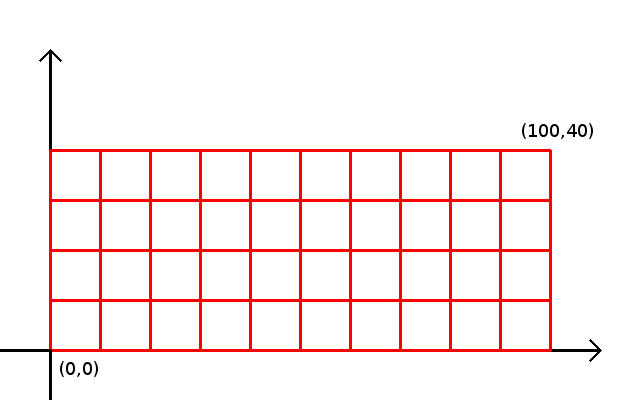(Rectangle starting at 0,0.)

But, we could also say that there are 100 smaller rectangles set side-by-side that are 40 units tall and 1 unit wide. If we step through each unit stack and add up their heights, we get 40 + 40 + 40… 100 times. This could be a simple empty-headed mindgame, except that this is how we could approximate the area of a less linear backyard, one that has been landscaped.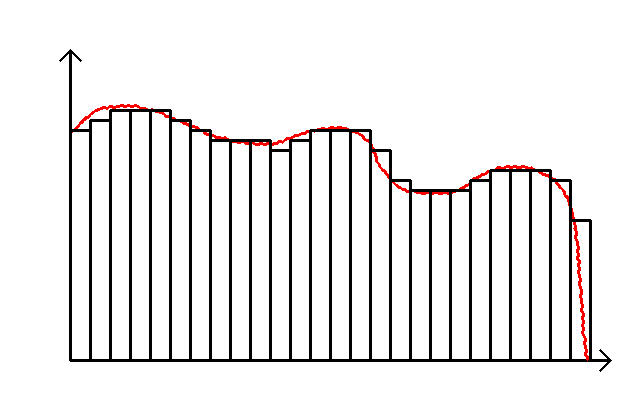(Finding the area of a backyard in Beverly Hills.)

We use the formula: area = sum(f(x)*delta x)

to represent this summation. This kind of looks like the Riemann Zeta function…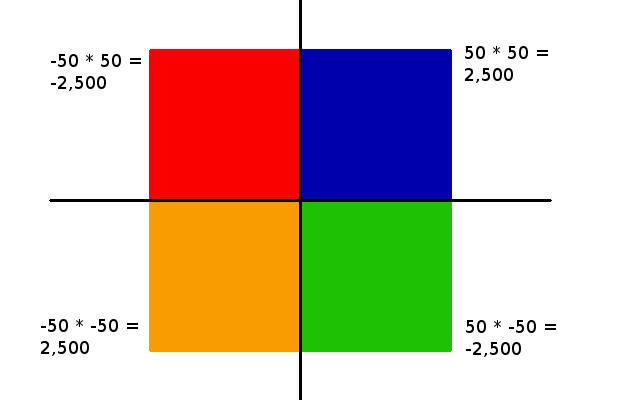(Identical squares located in each of the four quadrants.)

But, let’s get back to squares. It is possible to get a negative area. This can be thought of as removing dirt from a hole. In one sense, the removed dirt is positive, but in another, the hole is negative.

If you remember your multiplication properties:
positive times positive is positive
negative times negative is positive
negative times positive is negative
positive times negative is negative

Look at those squares. They’re the same square, just shifted into a different quadrant of the graph. What if there was something about the number -1 that had a magic ability to kick points around on the graph. Like, maybe that one corner at (0,0) was nailed in place and the square was rotating around the origin?

I was taught in school to write out points on a graph as (x0, y0). This is fine when you want to record the corner points of the backyard on a piece of paper, but it gets messy for anything more practical.

Rene Descartes gave us the cartesian coordinate system, which I’ve been using up to this point. In simple systems, the x-axis represents the variable in the formula that changes, while the y-axis is the output from the formula for that value of x.

y(x) = 2*x^2 + 3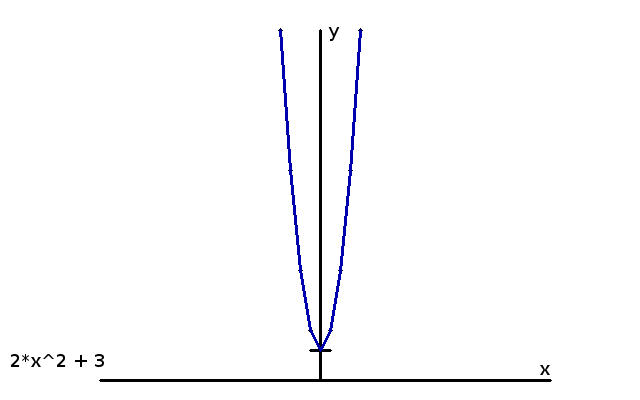(Graph where the vertical axis represents the output from f(x).

But, what if the part that changes has two movable parts? Such as changing “x” and “y” at the same time?

Multiplying x by a real number (any value between positive and negative infinity) acts like a scalar – it scales the size of x.

1 * 2 = (2, 3)
2 * 2 = (4, 3)
3 * 2 = (6, 3)

All we’re doing here is moving a point (x, 3) back and forth along the horizontal axis.Conversely, multiplying y by a real number still acts as a scalar – scaling the size of y.

1 * 3 = (2, 3)
2 * 3 = (2, 6)
3 * 3 = (2, 9)

This just moves the point (2, y) up and down along the vertical axis.

How can we change axes? How can we jump from the (x, 3) line to the (2, y) line?

# MMD Frame RendersI tried going back to the workfile from volume 5 to see if I could get MikuMikuDance to render the .avi for that. Unfortunately, I still got the “.dll file not found error”. I was thinking that it might be because of the way the vol. 6 project was set up (you’re given the main MMD project file already pre-assembled, then you have to browse to locate the Rana, guitar, stage and soundtrack files on your PC). Apparently not, though. I know that the installation worked at one time, because I rendered the full project back with volume 1.

Anyway, I then tried rendering a bitmap frame cap, and that worked. So, maybe my earlier problem with frame caps was due to the way lighting is defined in the vol. 6 demo project file. My only complaint is that there’s no way to specify the finished image size.So, this is what you’re working with for making music videos with Rana, so far.

# Riemann prose, part 4

So far, I’ve been treating numbers as if they are on a single straight line. As we increment the counting numbers, we move farther away from 0 to the right, but we’re stuck on the same line. This isn’t very useful if we want to know how big a pan we need if we’re making a sheet cake. We need to introduce a second line at right angles (90 degrees) to the first one. If the first line is our “x-axis”, then the second will be the “y-axis”.(Straight line, showing the zero crossing and the slope.)

Odds are that you saw the x-y axis in math class in school, and that it was used to graph equations like:

y = x + 1

This is a two-dimensional situation, and on an x-y graph, x is the part that changes from one point to another, and y is the result of the formula as x changes.

In this graph, x is infinitely continuous (no breaks or incremental steps) from negative infinity to positive infinity. There are two key elements in this formula:

Zero crossing
and the slope

If you have a straight line, you only need these two components to draw the line.

If y = 0, then x = -1, which gives us the zero-crossing.

Slope is the value multiplied against x. In my example, the slope is 1/1.

So, if we were to draw a line going through y=0 where x=-1, and go up one unit for every unit we go to the right, we’d have our graph without ever having to calculate any of the other points on the line.

One of the first things we look at with curves is where they cross y=0, and this is another important component of the Riemann Zeta function. But, more on that later.

Say we have a backyard, and we want to know how much sod to buy to re-sod it. What we could do is take a piece of graph paper and draw the yard on the x-y axes. It might look something like this: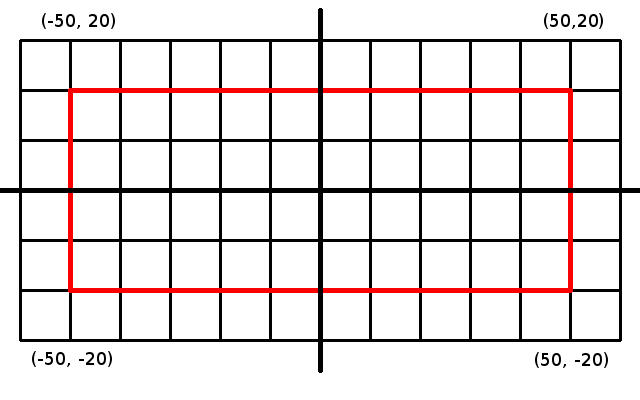(Backyard on graph paper.)

We start from the center of the yard and walk to the left, counting off feet, then turn left when we get to the edge and, starting from 0, count feet until we get to the far bottom corner. Maybe that was 50 feet left, and 20 down. We go back to the center and repeat, going to the left, but turning right at the edge and counting feet up from 0 again. So, 50 feet left and 20 feet up. Back to the center of the yard, and go right this time, giving us 50 feet right, 20 feet both up and down.

When I was a kid in school, we’d write these points out as (-50, -20), (-50, 20), (50, -20) and (50, 20), where the first value of the pair is x, and the second is y.

If we draw in the edges of the yard, we get a rectangle. Subtract the points from each other and we learn that our rectangle is 100 feet by 40 feet. To determine the amount of sod needed, we multiply the long side against the short side to get 4,000 square feet.

Odd… where did that “square” come from? I was using straight lines, and rectangles. Not no stupid squares…

# Bokaro P ni Naritai, vol. 6(Images used for review purposes only.)

I want to be a Vocaloid Producer, vol. 6, 1,500 yen, plus tax.

Time to set up a regular format for these blog entries. But first, some comments.

Tuesday Night:
I’ll be honest, these training DVDs aren’t the first thing I automatically run to when I get up out of bed in the morning. There’s a number of other things I’d rather be doing instead, or that I have no choice about. Not including my regular work, I’d gotten my hands on a couple Gameboy games, and that ate up 3-4 weeks of free time. Plus, I’d written up and illustrated the Riemann articles, created two animations for that and then came up with soundtracks, which took another few weeks. Then I accepted a part-time job to type up transcripts for a 3-hour business lecture in English, and a short trial project for doing an English rewrite, which combined represented a total of 60 more hours of work. In with all of this, I’ve been creating patterns in Gimp that I’ve been running on Facebook for the last 2-3 months.

What this means is that I’ve fallen behind on the Vocaloid tutorial videos. On Tuesday, I had a little extra time, so I sat down to finish the MMD tutorial from volume 4. This has been the most time-consuming one so far because I’m creating a walking cycle for Rana, and it takes several minutes to make each small move, then switch between MMD and the video to make sure I have the pose right.

MMD really is very powerful. If you select the right wrist and then pull it to the right, the bone structure is such that the upper body is pulled too, in a way that looks kind of natural. Lifting the right knee causes the upper thigh to rotate properly, and the lower leg and foot to lift the corresponding amount. But. While MMD does have English support for the menu items and buttons, the model components are mostly in untranslatable Japanese. So, I have to switch from Japanese to English to determine what the buttons are for, then back to Japanese to try to hunt for the model items “right hand” or “upper torso” prior to trying to position them.

Eventually, I did get halfway through the volume 4 tutorial, which consisted of raising the left arm and right leg in a marching pose. From here, you select the entire model, copy the current bone positions, select frame 10, and do a flip paste. This creates a key frame, but with the right arm and left leg raised. Select frame 20 and do a regular paste to make a second key frame that returns Rana to her pose in frame 0. Then, play the walk cycle in a loop. Somehow, MMD decided to reject the motions I’d registered for raising Rana’s legs and swing her arms properly. After 1-2 hours of work, I had nothing.

On the other hand, the tutorial goes on to say that Rana’s motion looks like she’s swimming through water and that there’s a lot of clean-up that needs to be done to fix things. The entire tutorial is about 9 minutes long, and I’m only at the 6 minute mark. Sigh. I’ve only briefly looked at the volume 5 MMD tutorial, and haven’t done anything with the volume 6 DVD at all.

Wednesday:

One of the things about stepping away from a problem is that almost immediately I started coming up with work-arounds. The tutorial occasionally reminds the viewer to click “Register” for the Bone Manipulation section, but I apparently missed a key point for this. Registering updates the current key frame (0 frame) only for the currently selected joint (right wrist, left ankle, neck), so EVERY time you move something, you have to register it before selecting the next joint. However, all of the positioning I did on Rana is still in effect if I save my work and reopen the file later. Couple this with the use of “V-Sel” (Select all joints) in the Frame Manipulation menu prior to doing the key frame copy-paste step, and maybe my fix would be to select all of the joints and then Register them in bulk. Using V-Sel only selects the registered joint positions. So, I tried using SHIFT-click to select a full range of joints at once, but this works more like CTRL-click in other programs (selecting only one item, not a range). Eventually, I selected all of the joints by SHIFT-clicking each one one at a time, and then clicking Register once for each block. So, yeah, now the walk cycle is broken exactly the same way as in the video.

The next step is to move the key frames for both feet so that they only move during a shorter period than the arms do, and add 2 new key frames so that Rana’s knees bend mid-step. After this, we drag and drop the “Rana theme music”, add a few blank frames to make the march line up with the music tempo, and finally copy-paste the walk cycle for the full length of the song. A possible bug I encountered here is that certain key points for specific joints don’t advance when a blank frame is inserted or deleted, and occasionally the copy-paste feature “loses” some of the selected key bone points. And, it took me a while to figure out how to delete unwanted key points that got pasted in the wrong place (rubber band select, or SHIFT-click select, then use the Frame Manipulation menu Delete button.)

Playback of the walk cycle matches the demo video. The tutorial suggests making small tweaks to have Rana’s mouth open and close to mimic singing, and you can make her eyes blink, if desired, as “self-study”. I didn’t bother with this part.

Once I had the vol. 4 MMD tutorial out of the way, I could move on to vol. 5. Here, the primary tasks are to import accessories and relate them to Rana. In the Model view, you can select specific accessories, such as the microphone or the dance stage, move them around, and change their size or transparency. For this tutorial, you import the Rana model from the vol. 1 DVD, and follow that with a pre-made vol. 5 motion file to give her the marching cycle again. There’s an interesting feature where you attach the mike to a specific joint of the Rana model, and any movement of that joint causes the mike to track appropriately. In this case, it’s Rana’s wrist. Use the pre-made pose off the DVD for Rana’s right hand half-open, and position the mike next to her palm, then individually curl her fingers so she’s kind of holding it right.

The idea is that she’s supposed to be singing in the mike, but the walk cycle has her swinging her arms, so you have to delete all of the key frames for her right arm and hand. When Rana is ready, delete all of the key frames for her main body position, import the dance stage, and move Rana as a unit up onto the stage. Whew. Done with this tutorial.

I’d jumped over the vol. 5 Vocaloid tutorial last time, so I came back to that, too. The focus is on Dynamics. This changes the pitch or energy of Rana’s voice for specific phonemes based on the current assigned note so that it sounds more human-like. As an example, say Rana is singing “la” for a full second. With a flat DYN pattern, the “La” sounds flat. Instead, you can draw a curve for DYN (which goes from 0 to 127) so that the “La” starts low, rises to a peak and then falls back down. This works just like the Pitch control from vol. 4. You can use straight lines or draw freehand with the pencil tool. That’s the entire tutorial.

Thursday:

Moved on to the vol. 6 DVD. See below.

New format for these blog entries
New magazine features:
Rana learns about the different parts of the guitar, and how to play simple chords. However, regular guitars are too large for her hands, so Robo-panda gives her a new accessory – White-kun, a voca-tar. Which she still can’t play. Then there’s the interview with Vocaloid Producer Mikito-P, an overview of the Chika Vocaloid model package, and a short write-up on the pick-up artist, Digital Sound Laboratory.

New DVD Features:
Video by Digital Sound Laboratory, entitled: Radiance of Nature
This is a kicking little piece that starts out with a lot of synth effects for the first minute, then settles into a pop-disco rhythm with a rather typical 80’s anime “looking to the future” lyric. Taken together it’s Jean Michel Jarre meets Macross. The video is just a still of Rana from one of the magazine covers, with a transparent “moving spotlights effect”. The music is good, but there’s nothing to the video, model animation-wise.

Rana’s voice samples:
Another 27 samples, laughing, talking and counting “1, 2 3”, with variations.(Extras page, with Chika, and Digital Sound Laboratory.)

Tutorials:
SSW:
Sing-Songer Writer has a large library of pre-written song examples, that it calls “arrangements,” for each genre. This includes Jazz, Cafe, Rock, Country, Fusion, etc. Each arrangement is made up of 2 to 5 instruments, and divided into “intro”, “A set”, “B set”, “Final” and “Closing”. The tutorial is to take the demo song and fill in the empty bars with parts of a specific arrangement. Some of the arrangement combinations come out pretty cool sounding, but I think this goes a long way in explaining why modern J-Pop has such a cookie cutter sound to it.

Vocaloid:
This time, it’s the Velocity parameter, which works the same way as Pitch and Dynamics. Velocity is that MIDI parameter that states how hard (or fast) a keyboard key was pressed. Because Japanese phonemes are generally a consonant-vowel pair (“ko” or “Ju”), [Vel] can be used to add stress to the consonant  portion, and have the stress drop off for the vowel portion.

MMD:
This one is a really good tutorial. I just wish it wasn’t so hard for me to follow. Steps: Import Rana, the guitar, the stage, Rana’s full motion data, and the .wav soundtrack. You now have a complete music video, with Rana singing, dancing and playing the guitar. Your task is to add the camera and lighting keyframes. I spent several hours trying to relate what the tutorial is talking about to the menu options. Eventually it did make sense, though.

Additional comments:
I was planning on doing some frame renders to demonstrate just how cool the entire model set looks, but for some reason, MMD just gives me black images. When I tried rendering to an .avi movie file, I was told that some .dll  can’t be found in the data directory. Because the required file is actually in the right location, I may have to uninstall and reinstall MMD. I’ll wait to do this until I have to enter the next registration number in a week or so.

# Riemann prose, part 3

I left off with the sentence about there being a lot of irrational numbers. This lets me segue into big numbers. Really big numbers! The ones that you can’t count on one hand big!

Say we have the equation:
x(n+1) = x(n) + 1
If we start at n = 0, and x0 = 0; then x1 = 0 + 1 = 1.

If we turn around and put 1 back into the formula,
x2 = x1 + 1 = 1 + 1 = 2
Put 2 back into the formula, we get 3! Where will the madness end!!?

x(n+1) = x(n) + 1 is unbounded. It just keeps getting bigger without limit.(Unbounded line going to infinity and beyond.)

By definition, this is what we (some of us; me) call “infinity” (∞). Infinity as a math concept was pretty much handed to us by Zeno around 450 BC. The symbol ∞, representing infinity, was popularized by John Wallis somewhere around the 1650’s AD. Euler, in the 1700’s, used “i” to mean “infinity”, kind of confusing things. So, let’s just ignore him.

There are different kinds of infinity, unless you consider the car brand, then there’s only one kind. But, for numbers, we have an infinite number of values between 0 and 1, giving us an infinite number of both rational and irrational numbers. There’s an infinite number of counting numbers (positive integers going from 1 to infinity). There’s an infinite number of prime numbers. We could say there’s an infinite number of negative integers, but maybe that’s just the positive integers facing the wrong direction on the graph.

Consider this,
x(n+1) = x(n) + 1
goes to infinity. (0, 1, 2, 3…)

x(n+1) = x(n) + 2
goes to infinity twice as fast. (0, 2, 4, 6…)

They both go to infinity, so does this mean that
x(n) + 2 = x(n) + 1
if we allow n (number of steps) to get big enough? Sadly, no, but it would be cool if it did.

This just means that infinity isn’t just one number, and we have to look at each formula on a case-by-case basis to determine what infinity is doing there.

x(n+1) = x(n) + 1
is unbounded. It just keeps getting bigger.

How about: y = 1/x ?

As x gets bigger, y gets smaller. y will approach 0 without ever actually getting there. Because x is unbounded and has no ultimate top value, 1/x will keep getting infinitely smaller. Conversely,

y = 1/0

Is undefined because this is in fact infinity, and thus has no upper value.

Does this mean that having infinity in a formula is a bad thing? Depends.

y = 1/((2 + 1/x))

As mentioned above, 1/x approaches 0 as x gets bigger. If we assume that x has gotten big enough, then:

y => 1/(2 + 0) => 1/2

Y converges to 0.5 as x approaches infinity. It doesn’t actually reach 1/2, since 1/x never actually gets to 0, but it comes close enough as to make no nevermind.

x = 0.5; y = 1/(2+2) = 0.25
x = 1; y = 1/(2 + 1/1) = 0.33
x = 2; y = 1/(2 + 0.5) = 0.40
x = 3; y = 1/(2 + 0.33) = 0.42
x = 4; y = 1/(2 + 0.25) = 0.44
x = 10; y = 1/(2 + 0.1) = 0.476
x = 100; y = 1/(2 + 0.01) = 0.498

So, infinity, as with fire, is our friend as long as we take precautions against being burned.

We’ll be seeing infinity showing up a lot as we go along, as well as convergence (in fact, we already saw (non-)convergence when we were introduced to the Riemann Zeta function).